# How Do You Read A SMD Resistor?

0
45### SMD Resistor Introduction

SMD Resistor, called Chip Resistor, is one type of resistors. It is a part made by mixing metal powder and glass glaze powder and printing on the substrate by screen printing. It is resistant to humidity and high temperature, and has a small temperature coefficient. Different resistors have different resistance values, for SMD resistors, how are these resistance values marked and read?

### What Do Markings on Resistors Mean?

Before acquiring how to read the resistance value, let us first understand how the resistance value is marked, including the four methods:
1. Direct marking method
Use numbers and unit symbols to mark the resistance value on the surface of the resistor, and the allowable error is directly expressed in percentage. If there is no deviation on the resistance, it usually takes ±20%.
2. Text symbol method
Use the regular combination of Arabic numerals and text symbols to indicate the nominal resistance value, and the allowable deviation is also represented by it. The number in front of the symbol indicates the resistance value of an integer, and the number after it indicates the resistance value of the first decimal place and the resistance value of the second decimal place in turn. Character symbols that indicate allowable errors: D-F-G-J-K-M allowable deviations are: ±0.5%, ±1%, ±2%, ±5%, ±10%, ±20%.
3. Digital method
A method of marking the nominal value with three digits on the resistor. The digits are from left to right, the first and second digits are effective values, and the third digit is the exponent, that is, the number of zeros, and the unit is Ω. Deviations are usually expressed in text symbols.
4. Color Code marking method
Mark the nominal resistance value and allowable deviation on the surface of the resistor with bands or dots of different colors, which is common in many regions.
Black-0, Brown-1, Red-2, Orange-3, Yellow-4
Green-5, Blue-6, Violet-7, Gray-8, White-9
Gold-±5%, Silver-±10 %, colorless-±20%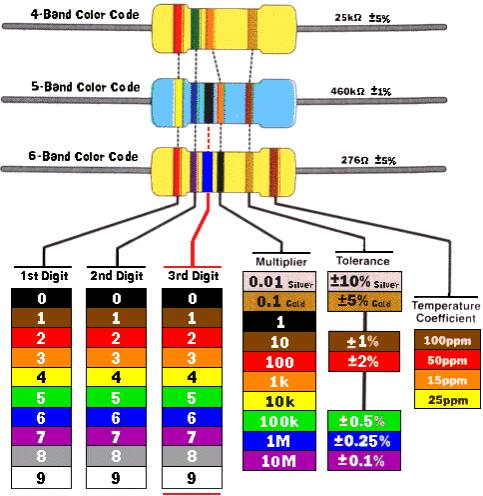For example, when the resistance is four rings, the last ring must be gold or silver, the first two digits are valid digits, the third digit is the power number, and the fourth digit is the deviation. When the resistance is five loops, the distance between the last loop and the front four loops is relatively large. The first three digits are significant digits, the fourth digit is the power number, and the fifth digit is the deviation.

### Resistance Value of the SMD Resistor

2.1 Character Code Marking
The resistance value of the chip resistor is the same as that of a general resistor, which is marked on the resistor body. There are three kinds of resistance nominal methods, but the nominal method is not exactly the same as that of general resistors. The surface of the usual chip resistors is marked with numbers, or represented by letters, and the resistance making method is as follows.
1. The first and second digits represent the significant number of resistance.
2. If the number at the beginning of the third digit is 0, it means tens of ohms (between 10 and 99 ohms). Examples, 100 is 10Ω, and 990 is 99Ω.
3. If the number starting with the third digit is 1, it means several hundred ohms (between 100 and 999 ohms). Examples: 101 is 100Ω, 151 is 150Ω, and 951 is 950Ω.
4. If the number at the beginning of the third digit is 2, it means several thousand ohms (between 1000 and 9999Ω). Examples: 102 is 1K, 152 is 1.5K, and 992 is 9.9K.
5. If the number starting in the third digit is 3, it means tens of K (between 10K and 99K). Examples: 103 is 10K, 223 is 22K, and 993 is 99K.
6. If the number starting in the third digit is 4, it means several hundred K (between 100K and 999K). Examples: 104 is 100K, 204 is 200K, and 854 is 850K.
7. If the number at the beginning of the third digit is 5, it represents a few M (between 1M and 9.9). Examples: 105 is 1M, 155 is 1.5M, and 955 is 9.5M.
8. If the number starting with the third digit is 6, it means ten M (between 100K and 999K). Examples: 106 is 10M, and 566 is 56M.
9. The standard method for four numbers is that the first three digits are real numbers, and the fourth digit is a multiple. Examples: 1001 is 1K, 1002 is 10K, and 1005 is 10M.
In addition, the chip resistors with packages 01005, 0201, and 0402 are too small in size, so there is no marked code, so they can only be measured and distinguished by a multimeter test.
2.2 Examples
1. With ±5% tolerance, the resistance code is three digits, and the nominal method of digital positioning is to use three digits on the resistor body to indicate its resistance. Its first and second digits are significant digits, and the third digit represents the number of “0”s added after the significant digits. No letters will represent something in this one.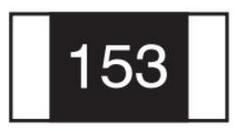153 = 15000Ω = 15KΩ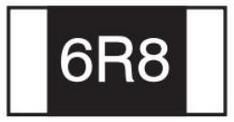≤10Ω: 6R8 = 6.8Ω (R represents a decimal point)

2. With package 0805, 1206, 1210, 2010, 2512, and tolerance ≤±1%, the resistor code has four digits. The first three digits are the effective digits of the resistance value, and the fourth digit means the number of zeros.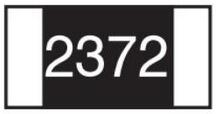2372 = 23700Ω = 23.7KΩ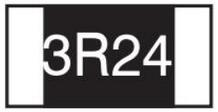≤10Ω: 3R24 = 3.24Ω (R represents a decimal point)

3. With package 0603 and tolerance ≤±1%, E-96 series standard resistance value, because the resistance body is too small, indicated with the three-digit resistance code (number) and the following index code (letter).
Index code (0603≤±1% mark)
Code: A-B-C-D-E-F-G-H-X-Y-Z
Index: 100–101–102–103–104–105–106–107–10-1–10-2–10-3
E-96 series standard resistance code (package 0603, tolerance ≤±1%)
The number code and letter mixed method also uses three digits to indicate the resistance value, that is, two digits plus one letter, and the letter represents the magnification.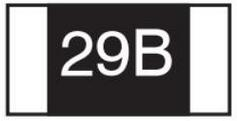1.96KΩ = 196×101Ω = 29B12.4Ω = 124×10-1 = 10X

With package 0603, tolerance ≤±1%, in the standard E-24 series, but not the E-96 series, the marking is the same as the 5% tolerance, but an extra line is added under the code.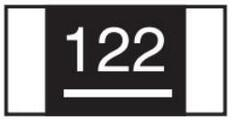122 = 1200 = 1.2KΩ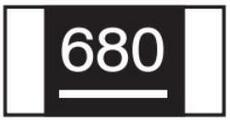680 = 38Ω
According to the above example of the resistance values of the SMD resistors, the resistance value of other chip resistors can also be obtained accordingly.

### SMD Resistor Value Judging Method

(1) Refer to the resistance value of the corresponding component in the same circuit.
In some electrical appliances, there are sometimes multiple circuits with the same structure, such as the backlight drive circuit of LCD color TVs, air conditioner inverter circuits, and so on. For a certain inverter circuit, the 4 IGBT drive branches are exactly the same. If a smd resistor in this part of the circuit is damaged, you can refer to the value of the chip resistors in other branches, namely R17=R51, R23=R48, R22=R49. If there is no mark, it can be measured and judged on the circuit board or the component can be removed for measurement.
In the same way, if the transistors, ICs or other components in the circuit are damaged, the parameters of the components can also be determined according to this method, for the replacement and repair.
(2) Determine the resistor parameters according to the circuit type.
In the MCU (microcontroller) circuit, some ports are often connected with pull-up or pull-down resistors. Ports connected with pull-up or pull-down resistors generally have an open-drain structure inside, which can avoid level drift in the I/O port and maintain a static and stable level. The resistance values of them are generally 10kΩ, 6.8Ω, 5.1kΩ, 4.7kΩ, 3.3kΩ, etc. If the value is too small, the power consumption will increase; if the value is too large, it will easily cause level drift or cause interfere. As long as it is determined that the damaged chip resistor is the pull-up or down-connect resistor of the MCU pin, it can be replaced with a resistor with a resistance value of 3.3 kΩ～10kΩ. Of course, it can also be replaced by referring to the resistance of other pull-up and pull-down resistors.
(3) Refer to similar models to determine resistance values.
If there is not the same circuit for reference, with the same type of model can be found, then comparative measurement can be carried out to determine the parameter value of the damaged component.
(4) The resistor value of the component obtained by the adjustment test.
If there is no similar model for reference, you can actually draw this part of the circuit at this time, determine the connection relationship of the damaged resistor in the circuit, and estimate its approximate resistance value based on the characteristics of the front and back circuits. If you are still unsure, use a large resistance potentiometer to replace the damaged resistor, adjust the potentiometer after power-on, and combine the key test point voltage of the circuit to roughly determine the resistance of the potentiometer.

### SMD Resistor Manufacturers/ Brands

What is the best resistor brand? Here is the list of world famous SMD Resistor manufacturers, including Bourns, IRC, KOA, ROHM, Ohmite, Panasonic, TE Connectivity, Vishay, Welwyn, YAGEO, TAI-I, WALSIN, LIZ, UNIOHM, FENGHUA, FOSAN, and so on.